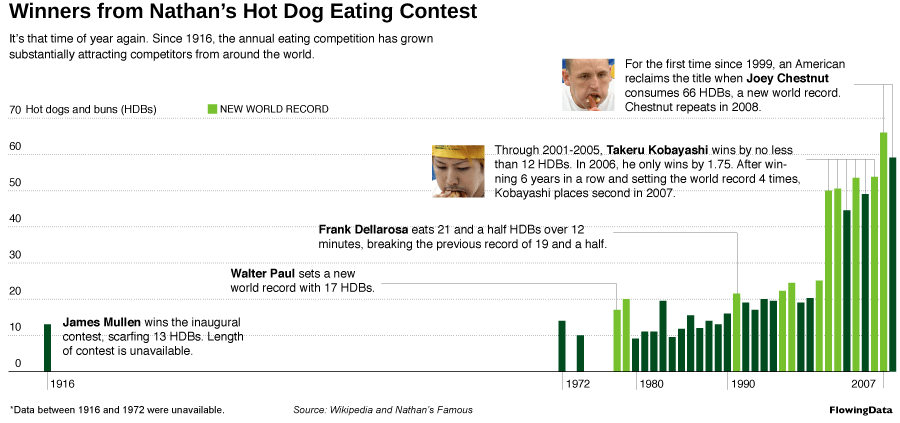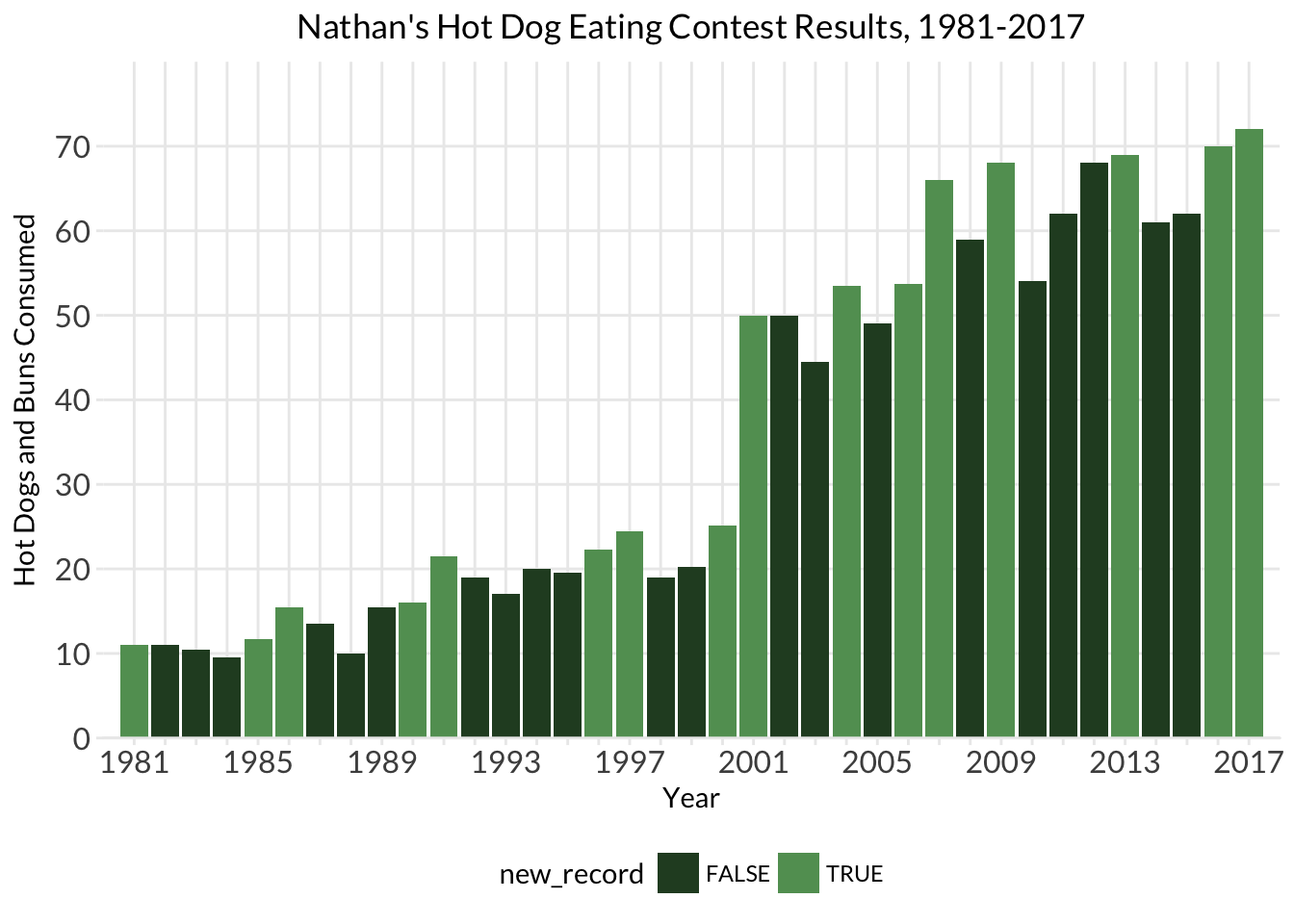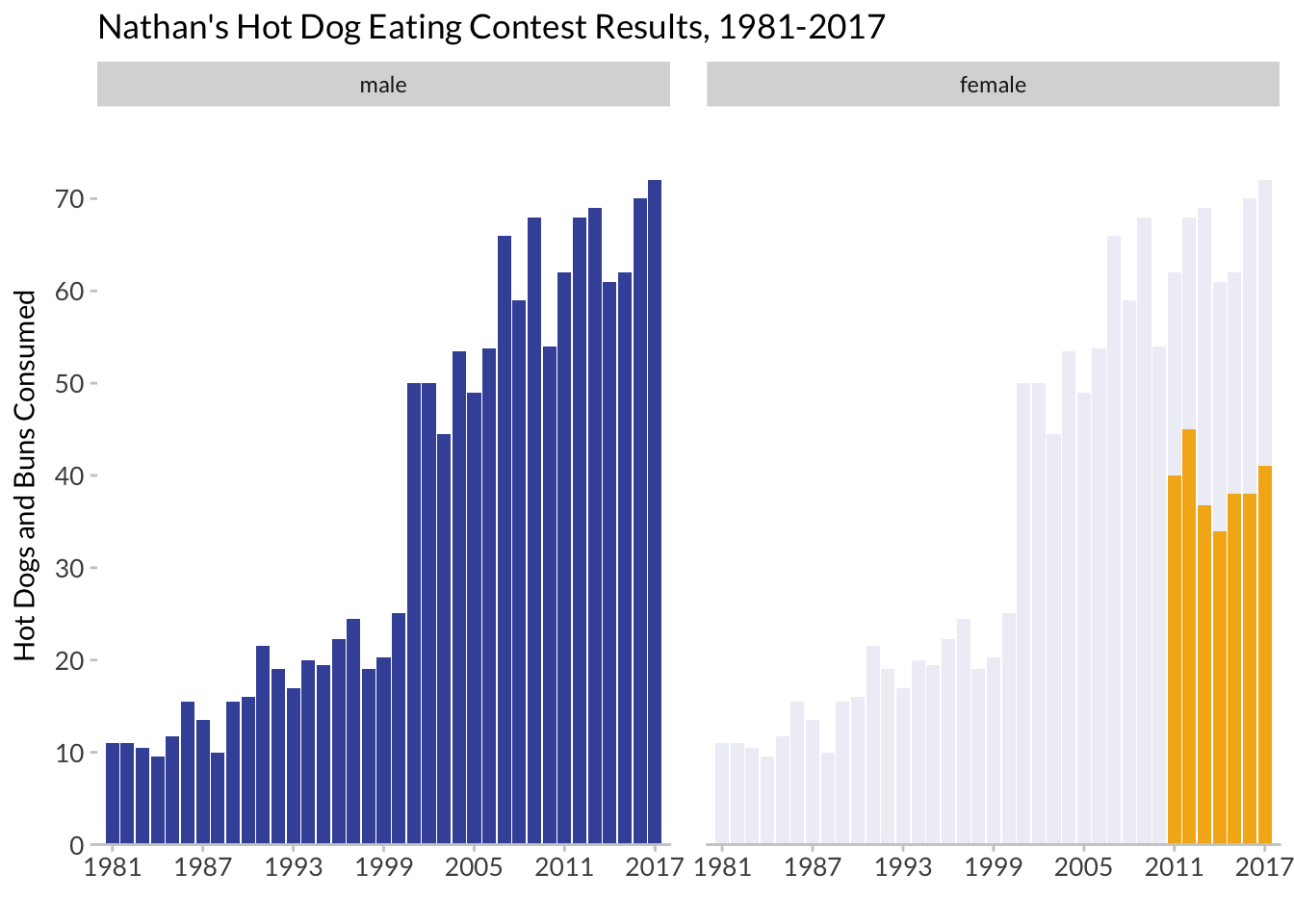# 1 Packages

library(tidyverse)
library(extrafont)
hot_dogs <- read_csv("http://bit.ly/cs631-hotdog",
col_types = cols(
gender = col_factor(levels = NULL)
))

# 2 Visualizing new world records (for males)Let’s adapt Nathan Yau’s hot dog contest example.

The first thing we notice is that we don’t have data about whether each year’s winner is a record or not. Since our data is nicely tidy, we can use dplyr window functions:

• First, we use base R’s cummax to create a new variable that reflects the maximum HDB eaten cumulatively, that is, compared to all earlier years. For this reason, the arrange(year) here is critical.

• Next, we want to know if the hdb_record is actually a new record or not, compared to all previous years. We can use case_when to create a logical variable that is TRUE if the hdb_record for a given year is greater than the hdb_record from the year before (using dplyr::lag). If not, this variable is FALSE.

hot_dogs_records <- hot_dogs  %>%
filter(year >= 1980 & gender == 'male') %>%
arrange(year) %>%
mutate(hdb_record = cummax(num_eaten),
new_record = case_when(
hdb_record > lag(hdb_record) ~ TRUE,
TRUE ~ FALSE
)) %>%
filter(year >= 1981)

We’ll also make our x-axis ticks again…

years_to_label <- seq(from = 1981, to = 2017, by = 4)
years_to_label
  1981 1985 1989 1993 1997 2001 2005 2009 2013 2017
hd_years <- hot_dogs_records %>%
distinct(year) %>%
mutate(year_lab = ifelse(year %in% years_to_label, year, ""))
hdb_records <- ggplot(hot_dogs_records,
aes(x = year, y = num_eaten)) +
geom_col(aes(fill = new_record)) +
labs(x = "Year", y = "Hot Dogs and Buns Consumed") +
ggtitle("Nathan's Hot Dog Eating Contest Results, 1981-2017") +
scale_fill_manual(values = c('#284a29', '#629d62')) +
scale_y_continuous(expand = c(0, 0),
breaks = seq(0, 70, 10)) +
scale_x_continuous(expand = c(0, 0),
breaks = hd_years$year, labels = hd_years$year_lab) +
coord_cartesian(xlim = c(1980, 2018), ylim = c(0, 80)) +
theme_minimal() +
theme(plot.title = element_text(hjust = 0.5),
axis.text = element_text(size = 12),
panel.background = element_blank(),
axis.line.x = element_line(color = "gray92",
size = 0.5),
axis.ticks = element_line(color = "gray92",
size = 0.5),
text = element_text(family = "Lato"),
legend.position = "bottom",
panel.grid.minor = element_blank())
hdb_records# 3 Visualizing background data

We’ll do this to highlight differences in gender.

https://drsimonj.svbtle.com/plotting-background-data-for-groups-with-ggplot2

hot_dogs_both <- hot_dogs %>%
filter(year >= 1981)
hot_dog_behind <- hot_dogs_both %>%
filter(gender == "male") %>%
select(-gender)
hdb_facets <- ggplot(hot_dogs_both,
aes(x = year, y = num_eaten)) +
geom_col(data = hot_dog_behind, fill = '#4254a7', alpha = .1) +
geom_col(aes(fill = gender), show.legend = FALSE) +
facet_wrap(~gender) +
labs(x = "", y = "Hot Dogs and Buns Consumed") +
ggtitle("Nathan's Hot Dog Eating Contest Results, 1981-2017") +
scale_fill_manual(values = c('#4254a7', '#f4b31a')) +
scale_y_continuous(expand = c(0, 0),
breaks = seq(0, 70, 10)) +
scale_x_continuous(expand = c(0, 0),
breaks = seq(1981, 2017, 6)) +
coord_cartesian(xlim = c(1980, 2018), ylim = c(0, 80)) +
theme(axis.text = element_text(size = 10),
panel.background = element_blank(),
axis.line.x = element_line(color = "grey80",
size = 0.5),
axis.ticks = element_line(color = "grey80",
size = 0.5),
text = element_text(family = "Lato"),
legend.position = "bottom",
panel.grid.minor = element_blank(),
panel.spacing = unit(1, "lines"))
hdb_facets